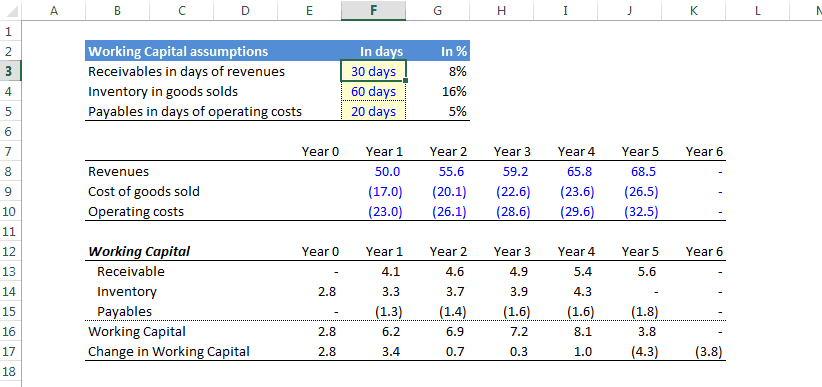top of page

## Coding

Working Capital

Modeling the working capital is important to make sure the financial model you have developped is not overly optimistic in assuming that all revenues are collected immediately and that all suppliers are paid immediately. The easiest way to model working capital is to assume a specific number of days of receivables, inventory and payables. These assumptions can be derived from historical analysis in the case of an existing project/company or by finding comparables for new projects.

Excel modeling

1. Specify the number of days of receivables, inventory and payables. Below is a suggestion for the units of each category:

a. Receivables: in days of turnover

b. Inventory: in days of cost of goods sold

c. Payables: in days of operating costs

2. Calculate the corresponding percentage of annual (if your model is annual) turnover, cost of goods sold and operating costs

3. Multiply the percentage calculated in 2 with the relevant reference (turnover, cost of goods sold, operating costs, etc.). For inventory it may make sense to multiply the number of days of cost of goods sold with the next period expected cost of goods sold as it is necessary to have the inventory before actually making the sales.In the example above it assumed that the project has a 5-year life and that inventory is calculated using the next year expected cost of goods sold.

bottom of page